python处理音频信号实战：手把手教你实现音乐流派分类和特征提取

1986年出版的《音乐心理学》一书中说到“人类和音乐遵循共同的规律”。研究发现，人类大脑的生理信号具有带直线区域的线性规律，在生理上具有普遍性，产生公式：S（f） 1 / f ɑ。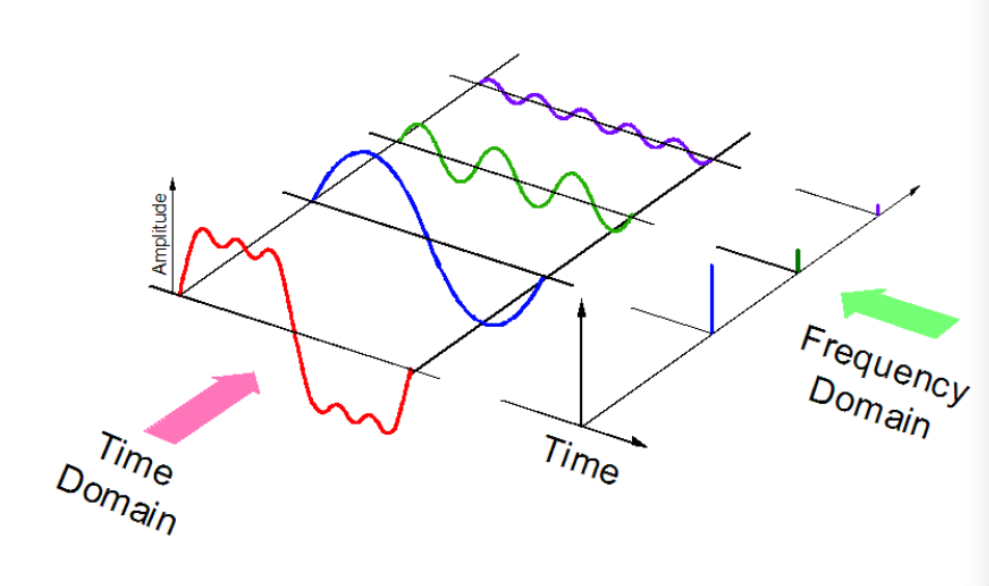• mp3格式
• WMA（Windows Media Audio）格式
• wav（波形音频文件）格式

Python有一些很棒的音频处理库，比如Librosa和PyAudio。还有一些内置的模块用于一些基本的音频功能。

1. Librosa

``pip install librosaorconda install -c conda-forge librosa``

2. IPython.display.Audio

IPython.display.Audio 让您直接在jupyter笔记本中播放音频。

``import librosaaudio_path = '../T08-violin.wav'x , sr = librosa.load(audio_path)print(type(x), type(sr))<class 'numpy.ndarray'> <class 'int'>print(x.shape, sr)(396688,) 22050``

``librosa.load(audio_path, sr=44100)``

``librosa.load(audio_path, sr=None)``

``import IPython.display as ipdipd.Audio(audio_path)````%matplotlib inlineimport matplotlib.pyplot as pltimport librosa.displayplt.figure(figsize=(14, 5))librosa.display.waveplot(x, sr=sr)``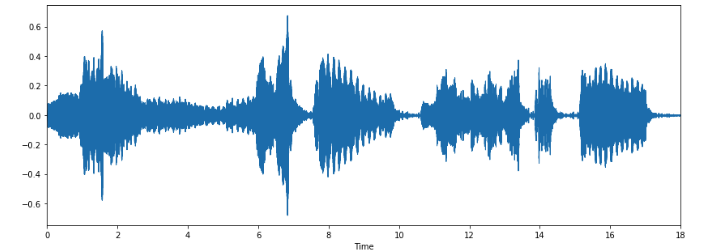#### 谱图

``X = librosa.stft(x)Xdb = librosa.amplitude_to_db(abs(X))plt.figure(figsize=(14, 5))librosa.display.specshow(Xdb, sr=sr, x_axis='time', y_axis='hz')plt.colorbar()``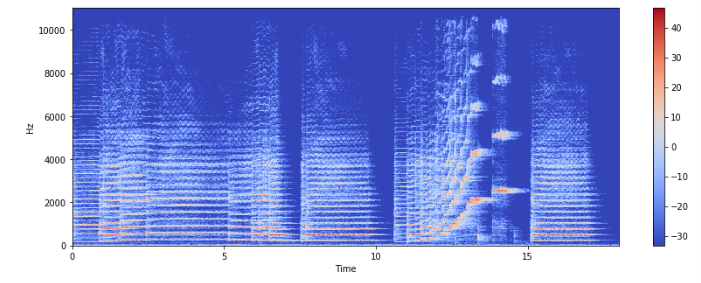``librosa.display.specshow(Xdb, sr=sr, x_axis='time', y_axis='log')plt.colorbar()``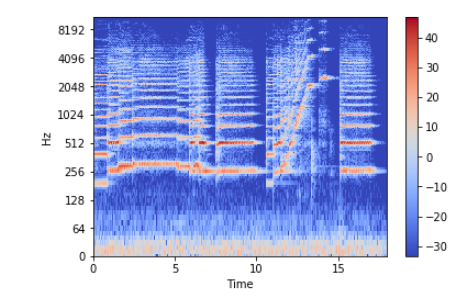librosa.output.write_wav 将NumPy数组保存到WAV文件。

``librosa.output.write_wav('example.wav', x, sr)``

``import numpy as npsr = 22050 # sample rateT = 5.0    # secondst = np.linspace(0, T, int(T*sr), endpoint=False) # time variablex = 0.5*np.sin(2*np.pi*220*t)# pure sine wave at 220 HzPlaying the audioipd.Audio(x, rate=sr) # load a NumPy arraySaving the audiolibrosa.output.write_wav('tone_220.wav', x, sr)``

``# Load the signalx, sr = librosa.load('../T08-violin.wav')#Plot the signal:plt.figure(figsize=(14, 5))librosa.display.waveplot(x, sr=sr)``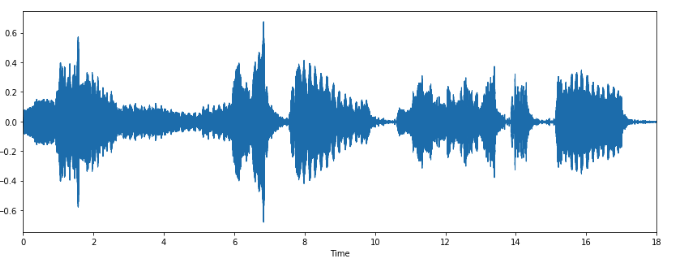``# Zooming inn0 = 9000n1 = 9100plt.figure(figsize=(14, 5))plt.plot(x[n0:n1])plt.grid()``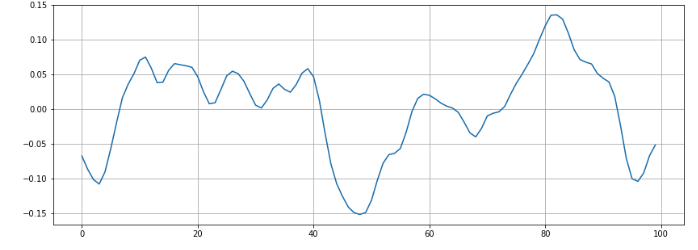``zero_crossings = librosa.zero_crossings(x[n0:n1], pad=False)print(sum(zero_crossings))6``

• 光谱质心

librosa.feature.spectral_centroid 计算信号中每帧的光谱质心：

``spectral_centroids = librosa.feature.spectral_centroid(x, sr=sr)spectral_centroids.shape(775,)# Computing the time variable for visualizationframes = range(len(spectral_centroids))t = librosa.frames_to_time(frames)# Normalising the spectral centroid for visualisationdef normalize(x, axis=0):    return sklearn.preprocessing.minmax_scale(x, axis=axis)#Plotting the Spectral Centroid along the waveformlibrosa.display.waveplot(x, sr=sr, alpha=0.4)plt.plot(t, normalize(spectral_centroids), color='r')``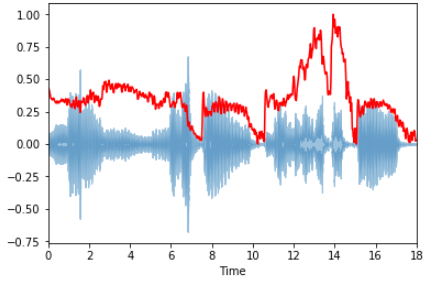• 光谱衰减

``spectral_rolloff = librosa.feature.spectral_rolloff(x+0.01, sr=sr)librosa.display.waveplot(x, sr=sr, alpha=0.4)plt.plot(t, normalize(spectral_rolloff), color='r')``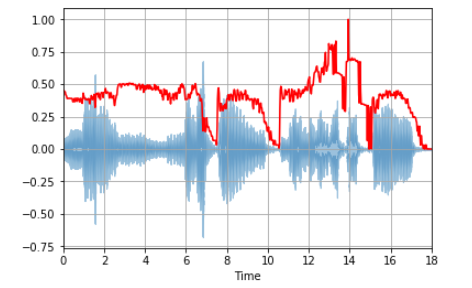• 梅尔频率倒谱系数``x, fs = librosa.load('../simple_loop.wav')librosa.display.waveplot(x, sr=sr)``

librosa.feature.mfcc 通过音频信号计算MFCC：

``mfccs = librosa.feature.mfcc(x, sr=fs)print mfccs.shape(20, 97)#Displaying  the MFCCs:librosa.display.specshow(mfccs, sr=sr, x_axis='time')``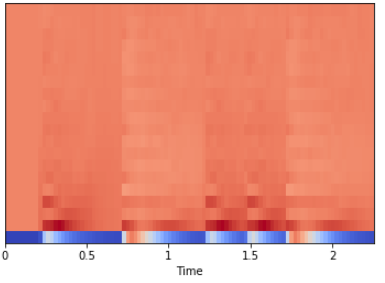``import sklearnmfccs = sklearn.preprocessing.scale(mfccs, axis=1)print(mfccs.mean(axis=1))print(mfccs.var(axis=1))librosa.display.specshow(mfccs, sr=sr, x_axis='time')``• 色度频率

``# Loadign the filex, sr = librosa.load('../simple_piano.wav')hop_length = 512chromagram = librosa.feature.chroma_stft(x, sr=sr, hop_length=hop_length)plt.figure(figsize=(15, 5))librosa.display.specshow(chromagram, x_axis='time', y_axis='chroma', hop_length=hop_length, cmap='coolwarm')``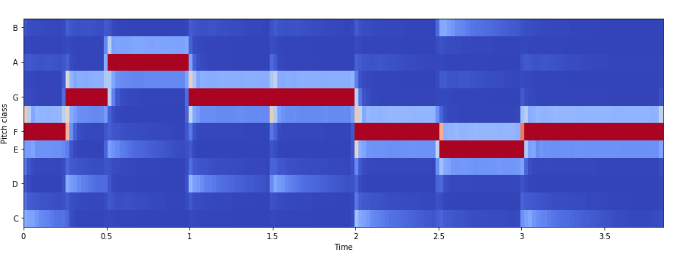``sox input.au output.wav``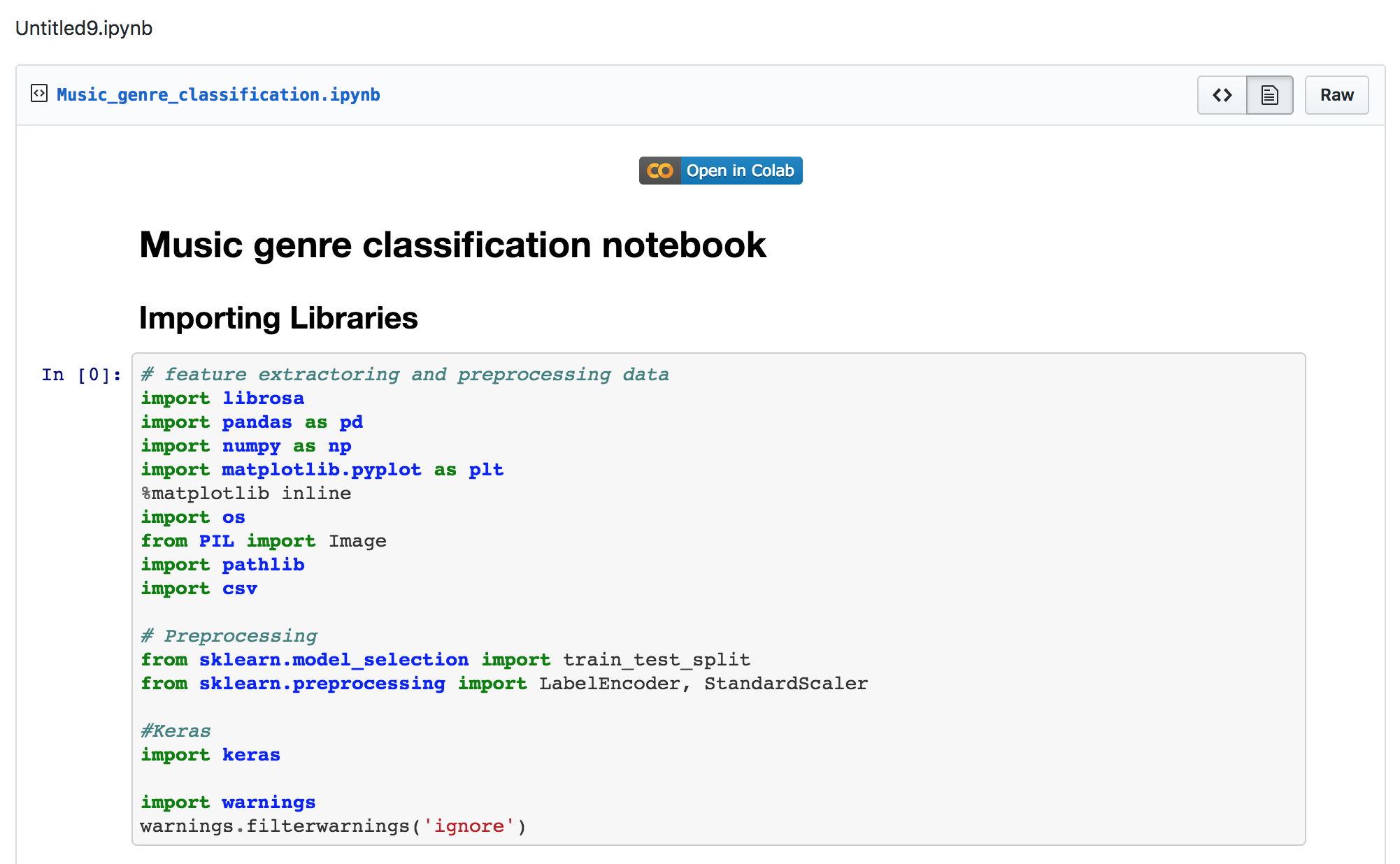[关于转载]：本文为AMiner唯一官方微信公众号学术头条原创文章。转载仅限全文转载并保留文章标题及内容不得删改、添加内容绕开原创保护且文章开头必须注明转自“SciTouTiao”微信公众号。谢谢您的合作。

[关于转载]：本文转载于学术君，仅用于学术分享，有任何问题请与我们联系：report@aminer.cn。